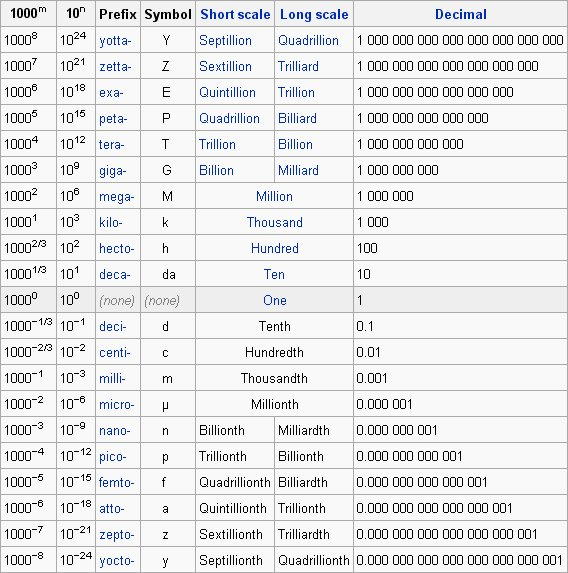### The International System (SI) of Units

Many general purpose spreadsheet and plotting tools are intended for business or financial applications and do not support the concept of scientific units; however, AfterMath is a tool designed to meet the needs of scientists and engineers, and one of its distinguishing features is the ability to keep track of proper scientific measurement units.

Internally, AfterMath explicitly associates all numeric data with a scientific unit derived from the International System of Units (SI System). This system is based on seven fundamental units of measure as shown in the table below:

Name Symbol Quantity
metre$m$ length
kilogram$kg$ mass
second$s$ time
ampere$A$ electric current
kelvin$K$ temperature
mole$mol$ amount of substance
candela$cd$ luminous intensity

AfterMath recognizes these seven fundamental units of measure and also many other units of measure which are derived from these seven units. These fundamental and derived SI units may be used in conjunction with the following standard metric prefixes.### Non SI UnitsThere are several units of measurement still in use by scientists and engineers which are not part of the SI system. AfterMath recognizes some (but not all) of these non-SI units. For example, the plot "Displacement vs Time" gives the distance in feet (an English unit) versus time in hours (rather than seconds). Even in this case, however, it is important to note that AfterMath stores the data internally in the appropriate SI units (meters and seconds). But for purposes of display, the data is automatically scaled to match the non-SI units that were chosen for this plot (feet and hours).

### Unitless Data

AfterMath will permit you to work with unitless data when necessary.